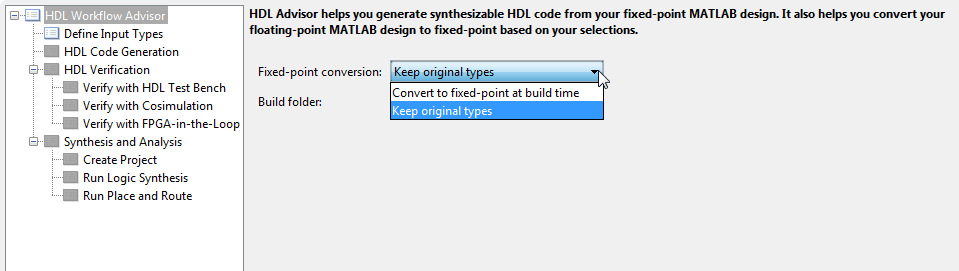# HDL Code Generation from A Non-Restoring Square Root System Object

This example shows how to check, generate and verify HDL code from MATLAB® code that instantiates a non-restoring square root system object.

### MATLAB Design

The MATLAB code used in this example is a non-restoring square root engine suitable for implementation in an FPGA or ASIC. The engine uses a multiplier-free minimal area implementation based on  decision convolutional decoding, implemented as a System object. This example also shows a MATLAB test bench that tests the engine.

```design_name = 'mlhdlc_sysobj_nonrestsqrt.m'; testbench_name = 'mlhdlc_sysobj_nonrestsqrt_tb.m'; sysobj_name = 'mlhdlc_msysobj_nonrestsqrt.m'; ```

Look at the MATLAB design.

```type(design_name); ```
```%%%%%%%%%%%%%%%%%%%%%%%%%%%%%%%%%%%%%%%%%%%%%%%%%%%%%%%%%%%%%%%%%%%%%%%%%% % MATLAB design: Non-restoring Square Root % % Key Design pattern covered in this example: % (1) Using a user-defined system object % (2) The 'step' method can be called only per system object in a design iteration %%%%%%%%%%%%%%%%%%%%%%%%%%%%%%%%%%%%%%%%%%%%%%%%%%%%%%%%%%%%%%%%%%%%%%%%%% function [Q_o,Vld_o] = mlhdlc_sysobj_nonrestsqrt(D_i, Init_i) % Copyright 2014-2015 The MathWorks, Inc. persistent hSqrt; if isempty(hSqrt) hSqrt = mlhdlc_msysobj_nonrestsqrt(); end [Q_o,Vld_o] = step(hSqrt, D_i,Init_i); end ```
```type(testbench_name); ```
```% Nonrestoring Squareroot Testbench % Copyright 2014-2015 The MathWorks, Inc. % Generate some random data rng('default'); % set the random number generator to a consistent state nsamp = 100; %number of samples nbits = 32; % fixed-point word length nfrac = 31; % fixed-point fraction length data_i = fi(rand(1,nsamp), numerictype(0,nbits,nfrac)); % clear any persistent variables in the HDL function clear mlhdlc_sysobj_nonrestsqrt % Determine the "golden" sqrt results data_go = sqrt(data_i); % Commands for the sqrt engine LOAD_DATA = true; CALC_DATA = false; % Pre-allocate the result array data_o = zeros(1,nsamp, 'like', data_go); % Load in a sample, then iterate until the results are ready cyc_cnt = 0; for i = 1:nsamp % Load the new sample into the sqrt engine [~, vld] = mlhdlc_sysobj_nonrestsqrt(data_i(i),LOAD_DATA); cyc_cnt = cyc_cnt + 1; while(vld == false) % Iterate until the result has been found [data_o(i), vld] = mlhdlc_sysobj_nonrestsqrt(data_i(i),CALC_DATA); cyc_cnt = cyc_cnt + 1; end end % find the integer representation of the result data idt = numerictype(0,ceil(nbits/2),0); % find the error in terms of integer bits ierr = abs(double(reinterpretcast(data_o,idt))-double(reinterpretcast(data_go,idt))); % find the error in terms of real-world values derr = abs(double(data_o)- double(data_go)); pct_err = 100*derr ./ double(data_go); fprintf('Maximum Error: %d (%0.3f %%)\n', max(derr), max(pct_err)); fprintf('Maximum Error (as unsigned integer): %d\n', max(ierr)); fprintf('Number of cycles: %d ( %d per sample)\n', cyc_cnt, cyc_cnt / nsamp); %EOF ```

### Create a New Folder and Copy Relevant Files

Execute the following lines of code to copy the necessary example files into a temporary folder.

```mlhdlc_demo_dir = fullfile(matlabroot, 'toolbox', 'hdlcoder', 'hdlcoderdemos', 'matlabhdlcoderdemos'); mlhdlc_temp_dir = [tempdir 'mlhdlc_so_nonrestsqrt']; % Create a temporary folder and copy the MATLAB files. cd(tempdir); [~, ~, ~] = rmdir(mlhdlc_temp_dir, 's'); mkdir(mlhdlc_temp_dir); cd(mlhdlc_temp_dir); copyfile(fullfile(mlhdlc_demo_dir, design_name), mlhdlc_temp_dir); copyfile(fullfile(mlhdlc_demo_dir, testbench_name), mlhdlc_temp_dir); copyfile(fullfile(mlhdlc_demo_dir, sysobj_name), mlhdlc_temp_dir); ```

### Simulate the Design

Simulate the design with the testbench prior to code generation to make sure there are no runtime errors.

```mlhdlc_sysobj_nonrestsqrt_tb ```
```Maximum Error: 3.051758e-05 (0.028 %) Maximum Error (as unsigned integer): 1 Number of cycles: 2000 ( 20 per sample) ```

### Hardware Implementation of the Non-Restoring Square Root Algorithm

This algorithm implements the square root operation in a minimal area by using a single adder/subtractor with no mux (compared to a restoring algorithm that requires a mux). The square root is calculated using a series of shifts and adds/subs, so uses no multipliers (compared to other implementations which require a multiplier).

The overall architecture of the algorithm is shown below, as described in .This implementation of the algorithm uses a minimal area approach that requires multiple cycles to calculate each result. The overall calculation time can be approximated as [Input Word Length / 2], with a few cycles of overhead to load the incoming data.

### Create a New HDL Coder Project

To create a new project, enter the following command:

```coder -hdlcoder -new mlhdlc_nonrestsqrt ```

Next, add the file `mlhdlc_sysobj_nonrestsqrt.m` to the project as the MATLAB function and `mlhdlc_sysobj_nonrestsqrt_tb.m` as the MATLAB test bench.

For a more complete tutorial on creating and populating MATLAB HDL Coder projects, see Get Started with MATLAB to HDL Workflow.

### Skip Fixed-Point Conversion

As the design is already in fixed-point, we do not need to perform automatic conversion.

Launch the HDL Advisor and choose `Keep original types` on the option Fixed-point conversion.### Run HDL Code Generation

Launch the Workflow Advisor. In the Workflow Advisor, right-click the Code Generation step and choose the option Run to selected task to run all the steps from the beginning through HDL code generation.

Examine the generated HDL code by clicking the links in the log window.

### Supported System objects

For a list of System objects supported for HDL code generation, see Predefined System Objects Supported for HDL Code Generation.

### Clean up the Generated Files

Run the following commands to clean up the temporary project folder.

```mlhdlc_demo_dir = fullfile(matlabroot, 'toolbox', 'hdlcoder', 'hdlcoderdemos', 'matlabhdlcoderdemos'); mlhdlc_temp_dir = [tempdir 'mlhdlc_so_nonrestsqrt']; clear mex; cd (mlhdlc_demo_dir); rmdir(mlhdlc_temp_dir, 's'); ```

### References

 Li, Y. and Chu, W. (1996) "A New Non-Restoring Square Root Algorithm and Its VLSI Implementations". IEEE International Conference on Computer Design: VLSI in Computers and Processors, ICCD '96 Austin, Texas USA (7-9 October, 1996), pp. 538-544. doi: 10.1109/ICCD.1996.563604